Einstein's Theory of Relativity

## Classical Mechanics

by   Paul Marmet
Chapter Five
of the Perihelion of Mercury.
5.1 - Mathematical Transformation of Units between Frames.
In this chapter we will deal with two kinds of transformations. The first kind is a mathematical transformation of units which brings no physical change to the quantities being described. In such a transformation, there is no physics, just mathematics. For example, let us suppose that we measure a rod on Mercury and find that it is 100 times longer than the local Mercury meter. Then we say that the length of the rod is 100 Mercury meters. However, if we know that on Mercury, the local meter is 1% longer than the local reference meter in outer space, we know that the same rod is actually equal to 101 times the outer space reference meter. These two descriptions by units of different frames are perfectly identical. The rod has not changed.
The observer on Mercury can also use his clock to measure a time interval. If the Mercury observer measures 100 units on his clock (i.e. 100 Mercury seconds), knowing that clocks on Mercury run at a rate which is 1% slower than clocks in outer space, we can calculate that during that absolute time interval the difference of clock displays on a clock in outer space will be 101 outer space units. No physics is involved in that transformation, only mathematics. The same physical phenomenon is described using different units.
Other units must also be transformed. For example, the absolute mass of the Sun does not change because we observe it from Mercury location near the Sun. However, measuring the same solar mass using the smaller Mercury unit of mass will lead to a larger number of Mercury units. Similarly, the physical amplitude of the absolute gravitational constant G does not change because the phenomenon takes place near the Sun. We have seen in chapter four that the absolute constant G is represented by different numbers of Mercury and outer space units. Again, no physics is involved.

5.1.1 - Consequence of a Simple Change of Units.
Let us suppose that using Newton's relationships, we want to calculate the period of Mercury using Mercury units. We must then compare this answer with the one obtained with the same relationships using outer space units. If we do so, we find that the numbers of units found for the period are different. However, when we take into account that the Mercury clock runs at a slower rate, we see that the absolute times obtained from either frame are the same.
In the next section we will see that in order to be compatible with the principle of mass-energy conservation, one must add another kind of transformations which are physical transformations. Contrary to the identical consequences resulting from the mathematical transformation explained above, different absolute results are found when Newton's laws are applied with the proper values belonging to different frames.

5.2 - Physical Transformations Due to Mass-Energy Conservation.
The second kind of transformations consists of real physical changes. We have seen in chapters one and three that when an object in outer space is moved to Mercury location, its absolute mass changes because of the change of gravitational potential and kinetic energies. (In the case of gravitational energy, the difference of mass is transformed into work). The object that remains at Mercury location is physically different from the object that existed in outer space because the dimensions of its atoms, their mass and clock rate have changed. This physical change of mass is quite different from the mathematical change of units mentioned above.
Here is an example. An observer on Mercury measures that a mass on his frame is 100 times larger that the unit of mass on Mercury. Another observer in outer space measures the mass of the same object after it has been carried out to outer space. In that new frame, the outer space observer finds the same number (100) of new units of mass. Both observers measure 100 local kilograms. However, the absolute mass of this object has changed when moved from Mercury location to outer space. The Mercury kilogram is not equal to the outer space kilogram. To realize this, we need to know the mass at Mercury location using outer space units. Applying the principle of mass-energy conservation, we find that using the same outer space units, the mass of the object is reduced to only 99 outer space kilograms when brought to Mercury location (since the Mercury kilogram is 1% lighter than the outer space kilogram). This is a real physical change. It is not a simple mathematical transformation of units like the one explained in section 5.1.
We will see in section 5.3 that these physical changes lead to results that are physically different when calculated using proper values in different frames. Using Newton's classical mechanics, we will find that the results obtained using the proper parameters in one frame are not coherent with the results obtained using parameters proper to another frame.
In order to clarify this description, in this chapter we will use the expression transformation of units to designate only a pure mathematical transformation of units. When a physical change is involved as a consequence of mass-energy conservation, we will speak of a transformation of parameters.
We consider that the interactions between physical elements (like fields, masses, lengths and clock rates) existing on Mercury, using Mercury parameters, must be the same as the ones that we calculate in outer space using outer space parameters. This means that the mathematical relationships so well-known in physics are the only ones that are valid but it is required that on Mercury we use the physical quantities (mass, length and clock rate) existing on Mercury while in outer space, we use the physical quantities (which are different) existing in outer space. In other words, we must always use proper values. It is totally illogical to use outer space physical parameters at Mercury location. On Mercury, we must necessarily use physical parameters that exist on Mercury.

5.3 - Incoherence between Outer Space and Mercury Predictions Using Newton's Physics.
In this book, we use Newton's equations which are always perfectly valid in all frames. However, there is a difference between Newton's equations and Newton's physics. Newton's physics is different from the physics described in this book because it is not compatible with the principle of mass-energy conservation. In Newton's physics, there is no place for changes of mass, length and clock rate. According to that physics, the mass of an object in outer space does not change if it is transported to Mercury location or to anywhere in the universe.
Let us suppose a Newtonian observer wants to measure the period of Mercury. He wishes to know its mass. To do this, he imagines the following thought experiment. He takes Mercury out of its orbit to outer space and puts the planet on a balance to measure its mass. Then he puts Mercury back on its orbit. Being a Newtonian observer using Newton's physics, the mass he will use in his calculations of Mercury's period will be the mass he just measured in outer space. However, we know this mass is wrong because of mass-energy conservation. We also know that other parameters (like length and clock rate) at Mercury location are modified due to the change of mass. Therefore this observer's Newtonian calculation of the orbit of Mercury will be wrong even when he uses the correct equations.
We will see that when the orbit of a planet moving around the Sun is calculated, using outer space physical parameters, we find a perfect ellipse. However, when we use the proper parameters existing on Mercury, we find a different orbit which is a precessing ellipse. This explains the advance of the perihelion of Mercury. When neglecting the changes of mass, length and clock rate on Mercury with respect to outer space, we find an erroneous prediction because we use outer space physical parameters instead of proper parameters.

5.4 - Incoherence of the Gravitational Force Using Newton's Physics.
Let us give an example that shows that the calculated force of gravity is different depending on what the physical parameters are used (outer space or Mercury). For the Newtonian observer, the gravitational force is:5.1
For that observer, whether the subscript of M(M) is o.s. or M makes no difference. We write o.s. because this observer uses Newton’s physics which always assumes a constant mass. The relevant physical parameters at Mercury location are: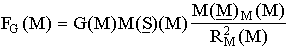5.2
All physical parameters in equation 5.2 must be Mercury physical parameters because that is where the interaction takes place. We will now compare these two equations. We know that the number of Mercury units to measure the mass of Mercury at Mercury location is the same as the number of outer space units to measure the mass of Mercury in outer space. This gives:
 M(M)M(M) = M(M)o.s.(o.s.) 5.3
The relationship between the number of units of mass of the Sun in outer space and Mercury units is given by equation 4.43:5.4
The relationship between the numbers of meters to measure the distance of Mercury from the Sun in outer space and Mercury units can be deduced from equation 4.34:5.5
Finally, the corresponding relationship for the gravitational constant G is given by equation 4.65: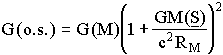5.6
Equations 5.3, 5.4, 5.5 and 5.6 in 5.2 give: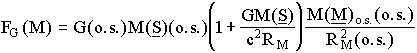5.7
In order to compare the gravitational force calculated using Mercury units, with the force calculated using outer space units, let us transform the number of units of force FG(M) into the corresponding number of outer space units. From equation 4.70, we have:5.8
Equation 5.7 with 5.8 gives:5.9
We must notice that equation 5.9 does not corresponds to a simple transformation of units. The physical parameters existing on Mercury at Mercury location have been taken into account.
Using the physical parameters existing on Mercury and outer space units, equation 5.9 shows that the absolute gravitational force on Mercury is different from the one calculated using the physical parameters existing in outer space and given in equation 5.1. The two results are not compatible. They predict different orbits. As explained above, the logical choice requires that we choose the equation obtained using the proper physical parameters existing at the location where the interaction of Mercury takes place with the gravitational field. We must reject the calculation obtained using outer space parameters when the experiment is taking place on Mercury. Finally, we now realize that equations 5.1 is the limit of equations 5.9 when RM®¥.
There is another direct consequence of mass-energy conservation. Contrary to equation 5.1, we see in equation 5.9 that using the physical parameters existing on Mercury, the decrease of the gravitational force is no longer perfectly quadratic. We will see in chapter six that in classical mechanics the orbits of an object submitted to a non quadratic gravitational force must have a precession.

5.5 - Relevant Physical Parameters.
Let us assume that an object on Mercury has a length of 100 Mercury meters. This means that independently of the units used to describe it, this is the relevant physical length. If we find that the meter on Mercury is 1% longer that the outer space meter, that length will be represented by 101 outer space meters. However, a Newtonian observer in outer space would predict 100 outer space meters from his own (incorrect) calculation.
In the case of time, if the Mercury observer measures that a phenomenon lasts 100 Mercury seconds, this means that the outer space observer measuring the same time interval on his clock (that runs 1% faster) will measure 101 outer space seconds. For the outer space observer, this means that the physics taking place on Mercury is such that the phenomenon takes place more slowly. We must remember that this is not a simple transformation of mathematical units. The difference is due to the slowing down of the processes on Mercury in order to maintain the internal coherence within the Mercury frame. One must recall that if the phenomenon takes place in outer space, the outer space observer will also measure 100 of his seconds which are different from 100 Mercury seconds. However, since the phenomenon is taking place on Mercury, it takes one extra outer space second before being completed.
If one could observe a physical phenomenon from outer space taking place in a very deep gravitational potential, one would see that objects are bigger and react more slowly. Furthermore if the outer space observer calculates quite independently the phenomena taking place on Mercury using outer space parameters, he would find that the observations reveal that everything functions at an unexpected slower rate with respect to his frame since the physics at Mercury location must be compatible with Newton's laws when using proper physical parameters.

5.6 - Fundamental Phenomena Responsible for the Advance of the Perihelion of Mercury.
This section is very important to understand the phenomena responsible for the advance of the perihelion of Mercury. Let us consider that the orbit calculated by the Mercury observer has a length equal to 1000 kilometers as determined with Newton's equations using proper parameters on Mercury. Of course, an observer located in outer space, also using Newton's equations and proper values existing in outer space will calculate that the length of the orbit is 1000 outer space kilometers.
Using mass-energy conservation, let us assume that due to a different gravitational potential, the unit meter on Mercury is 1% longer than the unit meter in outer space. Consequently, in order to be coherent, we calculate that clocks in outer space will run at a rate which is 1% faster than the rate on Mercury.
From the above information, let us calculate the clock display measured on the outer space clock DCD(o.s.) while Mercury travels the distance of 1000 kmM. Since the distance traveled is 1000 kmM, equation 4.34 shows that due to the longer Mercury meter, the outer space observer will measure 1010 kmo.s.. The circumference of the orbit is:

 Circ[M] = 1000 kmM = 1010 kmo.s. 5.1
This first correction on lengths ignores that while Mercury travels 1010 kmo.s. the clock in outer space runs 1% faster that the clock on Mercury. Since we must refer to the parameters existing on Mercury where the phenomenon takes place, the DCD on the outer space clock must be increased by one per cent with respect to the Mercury clock because of the faster rate of that outer space clock. Consequently, there is an increase of 1% of length to be traveled because the real length is 1010 kmo.s. plus another increase of 1% on the outer space clock because of its faster rate.
Consequently, in order to respect the physical laws existing on Mercury where the interaction with the gravitational potential takes place, we see that we must take into account two phenomena slowing down the completion of the ellipse in the frame where Mercury interacts with the gravitational potential. One is due to the increase of length of the Mercury meter and the second is due to the slowing down of the physical mechanisms on Mercury. We will calculate these two quantities in detail in the next sections of this chapter.
Let us note that in the above description, we have seen that the exact distance 1000 kmM (or 1010 kmo.s.) originally planned has been traveled as expected. However, we might calculate that the DCD(M) expected from calculations is different from the one measured. This is because not only Mercury, but also the clock has changed location (at a certain velocity) between the first and the last readings. This leads to a drift in the synchronization of the moving clock as explained clearly in sections 9.4, 9.5 and 9.6. The reading of chapter nine is necessary to complete the explanation on the loss of clock synchronization of moving clocks.

5.7 - Change of Length from Outer Space to Mercury Location.
We have seen that the relevant parameters responsible for the physical interaction with the solar gravitational field are the ones at Mercury location even though the final results are observed by the outer space observer. Let us calculate the physical length observed in outer space corresponding to the length calculated using Mercury parameters where the interaction takes place. There are two physical phenomena that make the Mercury meter longer than the outer space meter. The first one is due to the gravitational potential as explained in chapter one. The second phenomena is due to the velocity of Mercury on its orbit as calculated in chapter three.
Let aM(o.s.) and aM(M) be the numbers representing the semi-major axis of Mercury. Using equation 4.34, we get the relationship:5.11
Let us call lM(o.s.) the number of outer space meters for the length of Mercury's elliptical orbit and lM(M) the number of Mercury meters for the length of the same elliptical orbit. For a small eccentricity, lM(o.s.) is about 2paM(o.s.) and lM(M) is about 2paM(M). The eccentricity will be taken into account in section 5.10. We have from equation 5.11:5.12
We see in equation 5.12 that the number of meters measured by the observer in outer space for the length of the elliptical orbit is larger than the number of meters measured by the Mercury observer because the outer space meter is shorter.
Mercury is not only located in a gravitational potential, it also has a velocity. Because of this velocity v, there is a difference between the length of the moving meter and the length of the meter at rest, both at Mercury distance from the Sun (see equation 3.23). The moving Mercury meter is also the one that is relevant here since it is the one involved in the physics taking place on Mercury. The rest meter being shorter, the number of rest meters needed to describe the length of the orbit will be larger than the number of moving Mercury meters.
Let us call Nv the number of moving meters and No the number of rest meters to measure the Mercury orbit. Similarly to equations 4.30, 4.31 and 4.32, the absolute length L[rest] of the Mercury orbit is:
 L[rest] = No meter[rest] = Nv meter[mov] 5.13
where meter[rest] and meter[mov] represent respectively the length of a meter at rest and the length of a meter in motion. In equation 5.13, the absolute physical length L[rest] of the Mercury orbit does not change because we measure it with smaller meters at rest. Using equations 5.13 and 3.41 we have: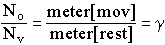5.14
which is:5.15
Using the first term of a series expansion gives:5.16
In order to calculate the velocity of Mercury on its orbit, let us use a well-known relationship in classical mechanics. The centrifugal force (C.F.) on a moving mass M(M) (Mercury) at a distance RM from the center of translation is equal to:5.17
In the case of a stable orbit around the Sun, the gravitational force F(grav) is equal to the centrifugal force. This gives:5.18
and5.19
Putting equation 5.19 in 5.16 gives:5.2
Equation 5.20 shows that the number of rest meters is larger than the number of moving meters.
Equation 5.12 gives the relative increase of the number of outer space meters with respect to the number of Mercury meters due to mass-energy conservation in the static gravitational potential of the Sun. Equation 5.20 gives another relative increase of the number of meters at rest with respect to the number of moving meters as explained in chapter three. From these two causes, the total relative number lo.s.,o of outer space meters at rest with respect to the moving Mercury meters is given by the product of equations 5.12 and 5.20. This gives:5.21
The first term of a series expansion gives: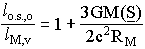5.22
which gives the total increase of distance in outer space units following the calculation of the length of the orbit using Mercury parameters, located in a gravitational potential at velocity v.

5.8 - Change of Clock Rate from Outer Space to Mercury Location.
There are two independent phenomena that slow down the clocks on Mercury's orbit. One is due to its gravitational potential, the other is due to its velocity. On the Mercury clock, during the period required to complete one full revolution, the difference of clock displays called DCDM(M) is smaller than the difference of clock displays DCDM(o.s.) in outer space since the physical mechanisms and clocks in outer space run at a faster rate. Let us calculate DCDM(o.s.) with respect to DCDM(M) during the same absolute time interval. From equation 4.49 we have:5.23
Let us now study the effect of velocity on clock rates. We have seen that due to mass-energy conservation, moving clocks are slower than clocks at rest. Using equation 3.10, we find: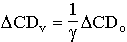5.24
where:5.25
DCDv is the difference of clock displays on a clock having a velocity v and DCDo is the corresponding difference of clock displays on a clock at rest (both clocks at the same distance from the Sun). Equations 5.24 and 5.25 give:5.26
Since v/c is very small with respect to unity, we consider the first term of a series expansion of equation 5.26. We get:5.27
or again,5.28
Equation 5.19 in 5.28 gives:5.29
The clock moving with Mercury is the one submitted to the interaction between the planet and the solar gravitational field. From equation 5.29, we see that the moving clock on Mercury runs more slowly than the clock at rest (at a constant distance from the Sun). Consequently, as explained above, the physical mechanism taking place at Mercury location is slower.
We have seen in equation 5.23 that clocks (and therefore the absolute physical mechanisms) slow down on Mercury as a consequence of the gravitational potential at that location. Equation 5.29 also shows a slowing down of the clocks due to the velocity of Mercury on its orbit. Let us calculate the total slowing down of clocks on Mercury due to both the gravitational potential and the velocity of Mercury on its orbit. The total difference of clock displays DCDM,v on moving Mercury with respect to the difference of clock displays DCDo.s.,o in outer space (at rest) is obtained using equations 5.23 and 5.29. We get:5.3
The first order gives:5.31
5.9 - Total Interaction Due to the Physical Changes of Length and Clock Rate.
We have seen in sections 5.7 and 5.8 how the changes of length and clock rate modify the period of translation of Mercury around the Sun. The first phenomenon given by equation 5.22 gives the relative length of the orbit as measured in outer space when the phenomenon is calculated using the parameters existing on Mercury where the interaction with the gravitational field of the Sun takes place. The circumference of the orbit lM,v using Mercury parameters corresponds to a longer length of the orbit as measured using outer space parameters. Therefore, the outer space observer will measure more than a full circumference using his own outer space units. Furthermore, we have seen in equation 5.31 that in order to be compatible with mass-energy conservation, clock rates and physical mechanisms taking place on Mercury must be slower than the ones measured in outer space. Consequently, it will take a larger number of seconds on the outer space clock to complete the circumference than on the Mercury clock.
Each phenomenon makes an independent contribution to modify lengths and clock rates on moving Mercury with respect to the ones at rest in outer space. Consequently both phenomena will contribute to the larger number of units for the period of Mercury as measured by an outer space observer.
Let us call Pl,DCD the period of the orbit of Mercury taking into account the combined effects of the change of length and the change of clock rate. In Pl,DCD(M,mov), "M,mov" is in round parentheses since Pl,DCD is a pure number without units. Then Pl,DCD(M,mov) is the number of Mercury units for completing the ellipse measured with a clock moving at velocity v at Mercury location and Pl,DCD(o.s.,rest) is the number of outer space units to complete the period of the ellipse measured with a clock in outer space having zero velocity. For clarity, we have dropped the subscript M indicating the location of the planet since we consider Mercury at its normal position in the Sun's gravitational field.
Let us add the contribution of the two phenomena described above. The correction on the period will be the product of the contributions given by equations 5.22 and 5.31. This gives:5.325.335.34
The first order gives: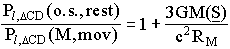5.35
Equation 5.35 shows that the number of units for the total period of Mercury is larger when measured using outer space units. Let us transform this result to calculate the relative increase of the period of Mercury as recorded by an observer using an outer space clock and an outer space meter. We find that the relative increase is given by the derivative of equation 5.35. This gives:5.36
Equation 5.36 shows that when Mercury has completed its full elliptical orbit, the observer using an outer space clock will monitor a period of translation larger by 3GM(S)/c2RM times Pl,DCD (M,mov).
Before completing this section, we must notice that following Newton's law, the advance of the perihelion of Mercury given by equation 5.36 can be written in a more simple form. Let us consider the gravitational potential "Pot" as a function of the distance RM from the Sun. Contrary to the definition of potential in electricity, in mechanics the potential is defined as the energy. Let us consider the energy per unit of mass. Using Newton's law of gravitation, we see that this ratio (which corresponds to the concept of potential in electricity) is independent of the mass of Mercury. Writing differently Newton's law we find that the gravitational potential is: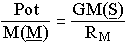5.37
Combining 5.36 with 5.37, we get:5.38
Equation 5.38 shows that the total advance of the perihelion of Mercury depends only on the constant 3/c2 times the change of gravitational energy per unit of mass. Equation 5.38 takes into account both the gravitational potential and the velocity of Mercury.

5.10 - Correction for an Elliptical Orbit.
There is one more term that needs to be taken into account to get a better accuracy. We know that Mercury travels on an elliptical orbit. However, in our calculation we have always considered the distance of Mercury from the Sun (RM) as a constant. In an elliptical motion, the distance from the Sun is not constant but varies according to a relationship characteristic of an ellipse. From geometrical considerations, it is demonstrated  that the distance RM of the orbiting body (Mercury) from the occupied focus (where the Sun is located) of an ellipse is given by the relationship: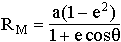5.39
where a is the length of the semi-major axis, e is the eccentricity of the ellipse and q is the angle between the value of the perihelion minus the argument of the perihelion. From equation 5.39, we see that when the eccentricity e is equal to zero, the distance of the orbiting planet to its center of translation is equal to a constant "a". Therefore equation 5.36 is valid when the eccentricity of the orbit of the planet is zero (or negligible). This is not the case for Mercury for which the eccentricity is e = 0.2056.

The orbiting body is sometimes at a closer distance from the Sun where the gravitational potential is larger. At those times, the velocity of the planet is larger. Of course, there are other parts of the orbit where the planet moves more slowly in a shallower gravitational potential. However, we can see that the smaller gravitational potential does not compensate completely for the larger one. The eccentricity must be taken into account. The clock rate and the unit of length must be taken into account at every point of the elliptical orbit. We have calculated above that the change of gravitational potential and of velocity produce an average effect represented mathematically by an "effective potential" Pot/M(M) in equation 5.38. Combining equations 5.39 and 5.37 we find:5.4
Equation 5.40 shows that the potential per unit of mass is not constant during an elliptical orbit (contrary to a circular orbit). Therefore the advance of the perihelion of Mercury after a full translation depends on the integral of that potential (Pot/M(M)) over a full translation of Mercury around the Sun. This integral gives the average equivalent gravitational potential during a full elliptical orbit. It is equal to 1/2p of the integral of the angle q over 2p. Using equation 5.40, we get: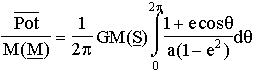5.41
This gives:5.42
The average gravitational potential obtained when the eccentricity eM for Mercury is:5.43
The average of Pot/M(M) gives the correction to Mercury's elliptical orbit with respect to a circular orbit. In order to apply that correction, let us substitute the equivalent potential of Mercury by the average potential given by equation 5.43. Equation 5.43 into 5.38 gives:5.44
Equation 5.44 shows that an outer space clock takes an extra fraction of a circumference to complete the ellipse when corrections include ellipticity. This extra fraction of a circumference D(circ) per unit circumference is: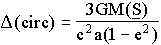5.45
Equation 5.45 is usually presented in radians instead of a fraction of a circumference. If the advance of the perihelion is represented by the angle Df, equation 5.45 becomes 2p times larger and gives:5.46
Equation 5.46 is the final equation for the advance of the perihelion of Mercury in radians per translation of Mercury as calculated using classical mechanics and mass-energy conservation.

5.11 - Mathematical Identity with Einstein's Equation.
Einstein presented a mathematical relationship for the advance of the perihelion of Mercury. Many books report that result. Straumann's  equations 3.1.11 and 3.3.7 give:5.47
This equation is perfectly identical to our equation 5.46. Consequently, all the physical principles that have been used to find equation 5.46 are sufficient since we get a prediction identical to the experimental observations and Einstein's equation. We add that the experimental value for the advance of the perihelion of Mercury has been well-known for more than a century. Le Verrier's calculations of the observational data found such an advance as early as 1859 . Roseveare published a very interesting historical account of reliable observations and calculations of Mercury's perihelion .

5.12 - References.

 Kenneth R. Lang, Astrophysical Formulae, Springer-Verlag, ISBN 3-540-09933-6. second corrected and enlarged edition, p. 541, 1980.

 Norbert Straumann, General Relativity and Relativistic Astrophysics, Springler-Verlag, second printing, 1991.

 U. J. J. Le Verrier, Théorie du mouvement de Mercure, Ann. Observ. imp. Paris (Mémoires) 5, p. 1 to 196, 1859.

 N. T. Roseveare, Mercury's Perihelion from Le Verrier to Einstein, Clarendon Press, Oxford, 208 p. 1982.

5.13 - Symbols and Variables

 aM(M) number of Mercury meters for the semi-major axis aM(o.s.) number of outer space meters for the semi-major axis DCDM(M) DCD for the period of Mercury measured by a Mercury clock DCDM(o.s.) DCD for the period of Mercury measured by an outer space clock DCDM,v DCD for the period of Mercury measured by a moving Mercury clock DCDo.s.,o DCD for the period of Mercury measured by an outer space clock at rest DCDo DCD for the period of Mercury on a clock at rest DCDv DCD for the period of Mercury on a clock in motion DPl,DCD(o.s.,rest) relative increase of the number of absolute seconds for the period of Mercury Df advance of the perihelion of Mercury in radians FG(M) number of Mercury newtons for the gravitational force on Mercury FG(o.s.) number of outer space newtons for the gravitational force on Mercury G(M) number of Mercury units for the gravitational constant G(o.s.) number of outer space units for the gravitational constant kmframe length of the local kilometer in a frame lM(M) number of Mercury meters for the orbit of Mercury lM(o.s.) number of outer space meters for the orbit of Mercury lM,v number of Mercury moving meters for the orbit of Mercury lo.s.,o number of outer space rest meters for the orbit of Mercury L[rest] length of the orbit of Mercury in rest units meter[frame] length of the local meter in a frame M(M)M(M) number of Mercury kilograms for Mercury at Mercury location M(M)o.s.(o.s.) number of outer space kilograms for Mercury in outer space M(S)(M) number of Mercury units for the mass of the Sun M(S)(o.s.) number of outer space units for the mass of the Sun No number of rest meters for the orbit of Mercury Nv number of moving meters for the orbit of Mercury Pl,DCD(o.s.,rest) number of outer space (rest) seconds for the period of Mercury taking into account the gravitational potential and the velocity of Mercury Pl,DCD(M,mov) number of Mercury (motion) seconds for the period of Mercury taking into account the gravitational potential and the velocity of Mercury RM(M) distance of Mercury from the Sun in Mercury units RM(o.s.) distance of Mercury from the Sun in outer space units
<><><><><><><><><><><><>
Chapter4 Contents      Chapter 6